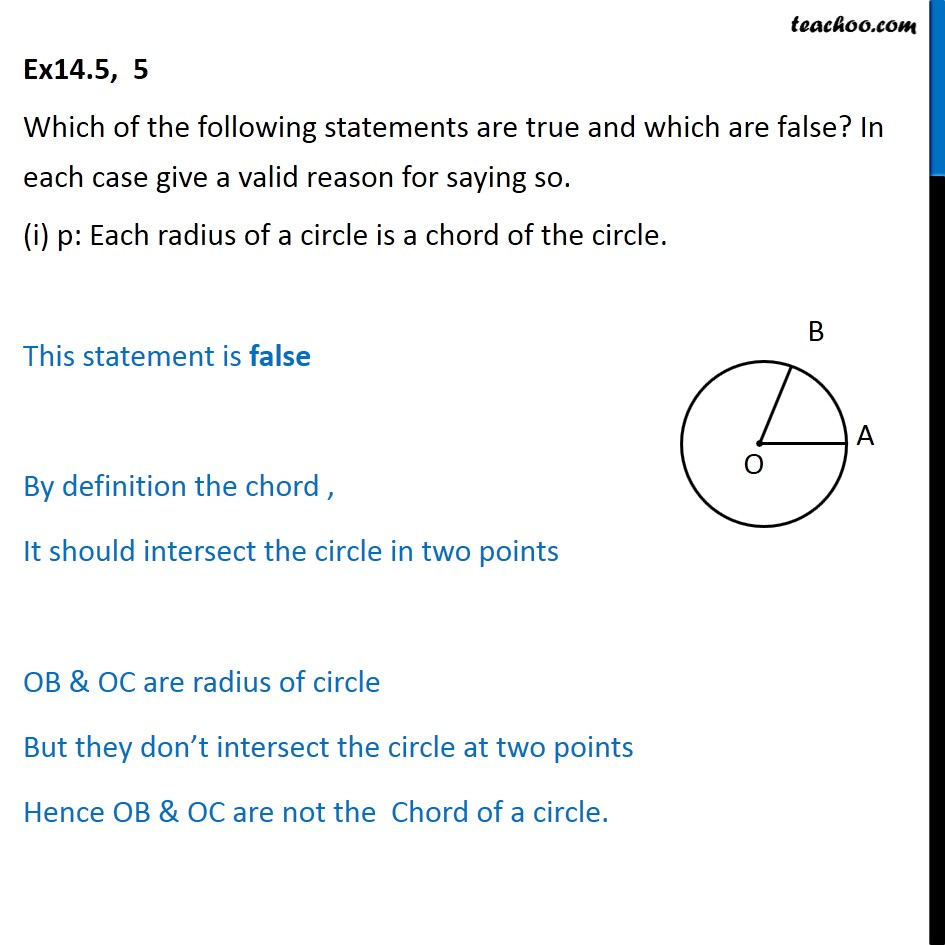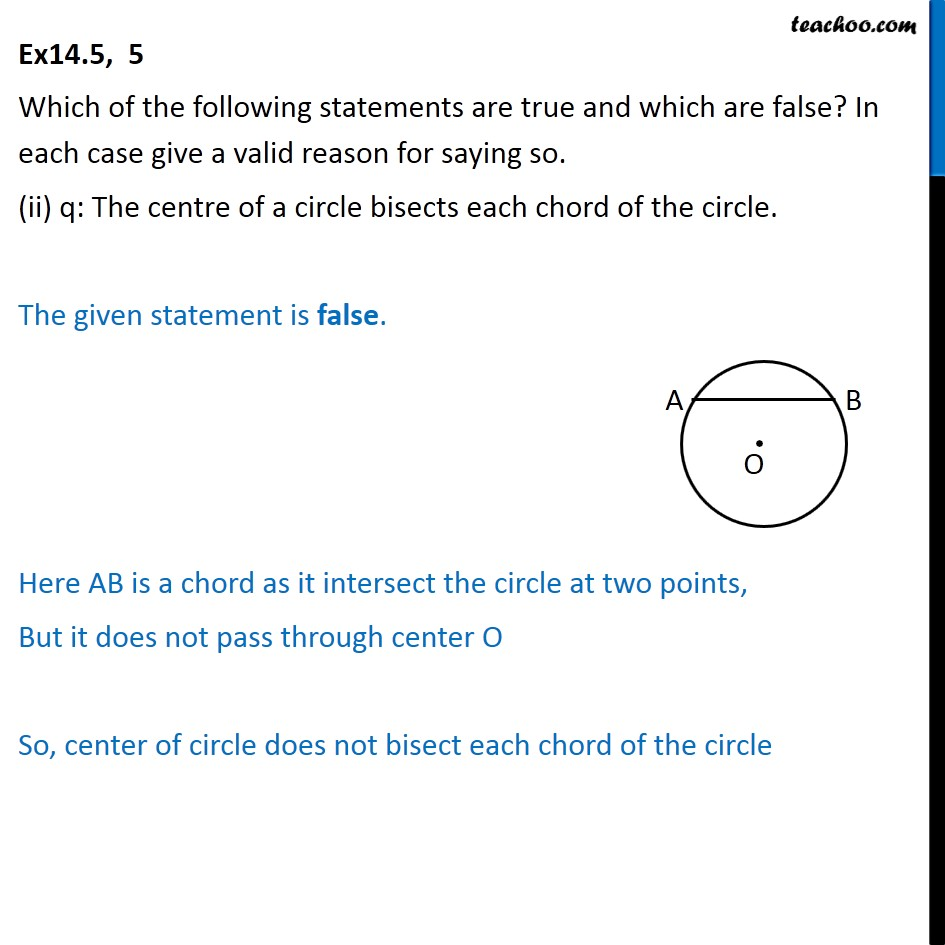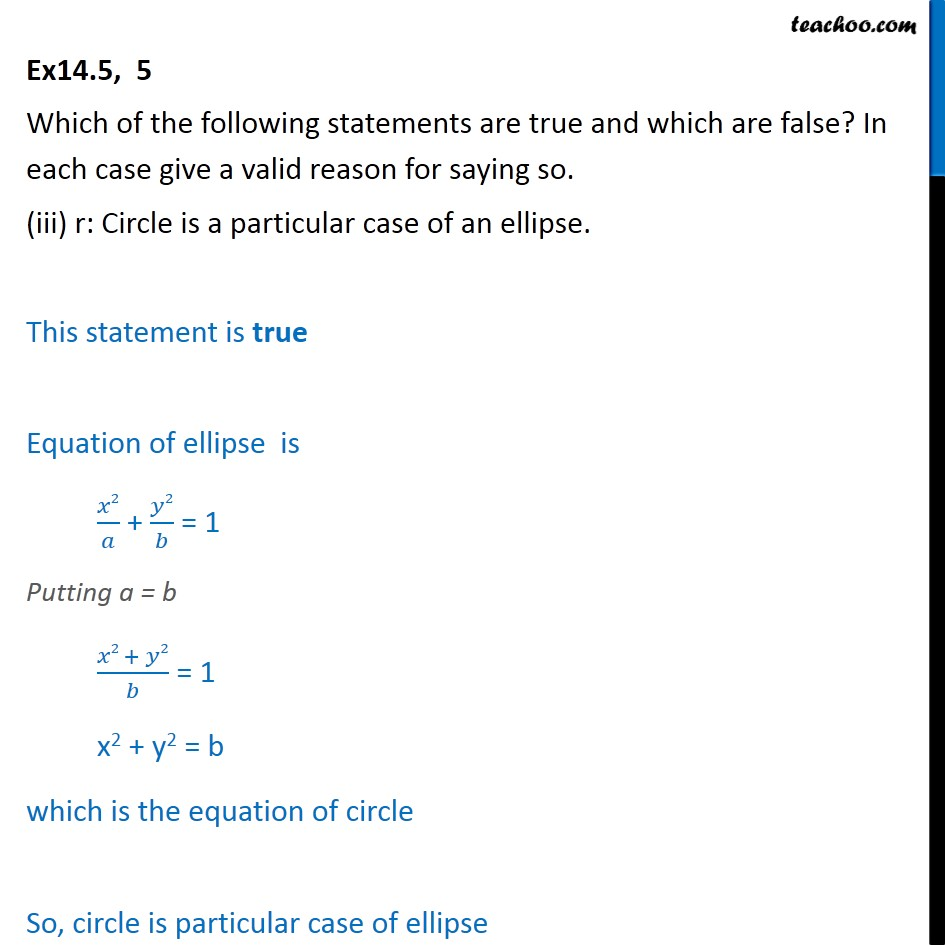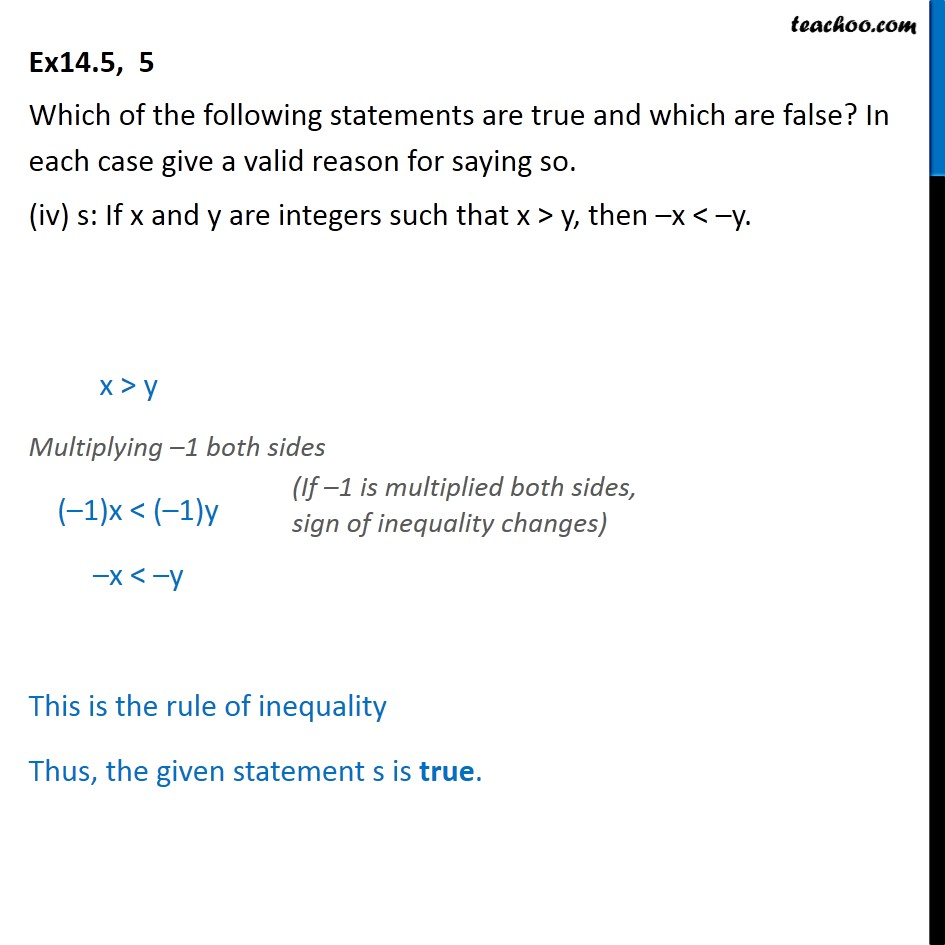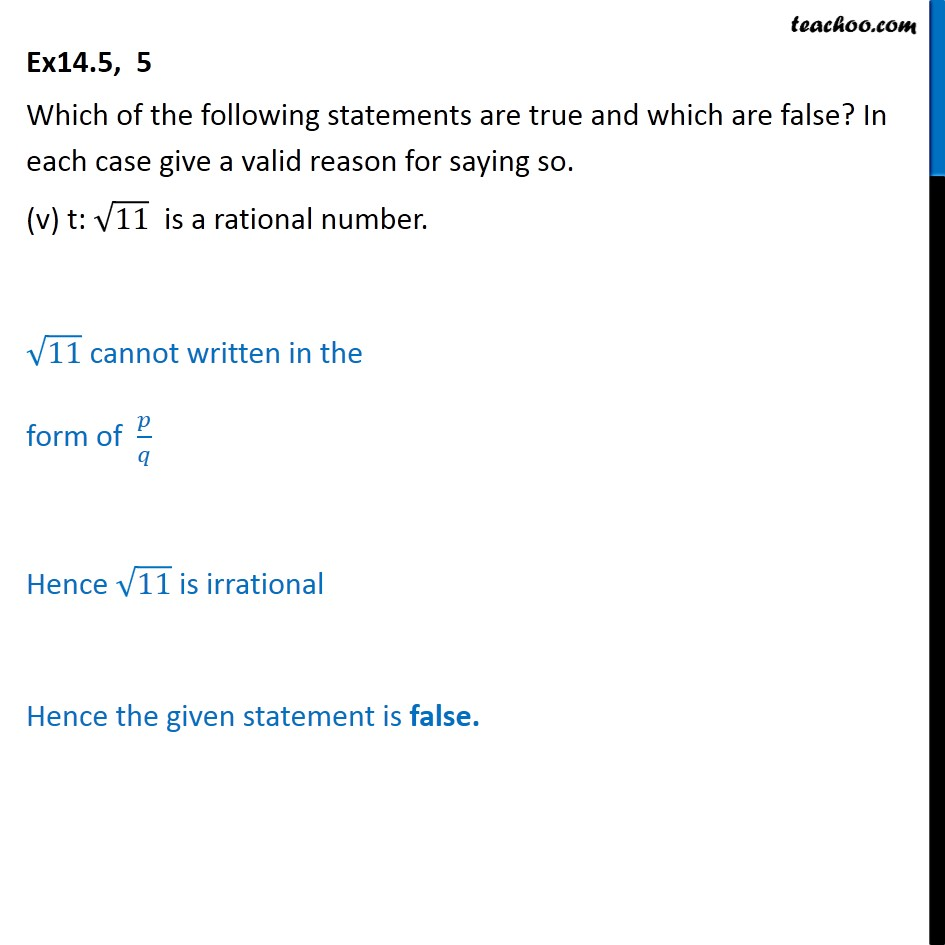Subscribe to our Youtube Channel - https://you.tube/teachoo

1. Chapter 14 Class 11 Mathematical Reasoning
2. Serial order wise
3. Ex 14.5

Transcript

Ex 14.5, 5 Which of the following statements are true and which are false? In each case give a valid reason for saying so. (i) p: Each radius of a circle is a chord of the circle. This statement is false By definition the chord , It should intersect the circle in two points OB & OC are radius of circle But they don’t intersect the circle at two points Hence OB & OC are not the Chord of a circle. Ex14.5, 5 Which of the following statements are true and which are false? In each case give a valid reason for saying so. (ii) q: The centre of a circle bisects each chord of the circle. The given statement is false. Here AB is a chord as it intersect the circle at two points, But it does not pass through center O So, center of circle does not bisect each chord of the circle Ex14.5, 5 Which of the following statements are true and which are false? In each case give a valid reason for saying so. (iii) r: Circle is a particular case of an ellipse. This statement is true Equation of ellipse is ﷐𝑥2﷮𝑎﷯ + ﷐𝑦2﷮𝑏﷯ = 1 Putting a = b ﷐𝑥2 + 𝑦2﷮𝑏﷯ = 1 x2 + y2 = b which is the equation of circle So, circle is particular case of ellipse Ex14.5, 5 Which of the following statements are true and which are false? In each case give a valid reason for saying so. (iv) s: If x and y are integers such that x > y, then –x < –y. x > y Multiplying –1 both sides (–1)x < (–1)y –x < –y This is the rule of inequality Thus, the given statement s is true. Ex14.5, 5 Which of the following statements are true and which are false? In each case give a valid reason for saying so. (v) t: ﷐﷮11﷯ is a rational number. ﷐﷮11﷯ cannot written in the form of ﷐𝑝﷮𝑞﷯ Hence ﷐﷮11﷯ is irrational Hence the given statement is false.

Ex 14.5